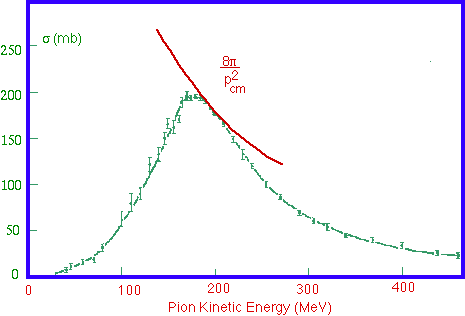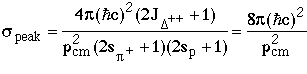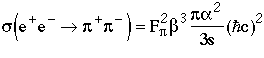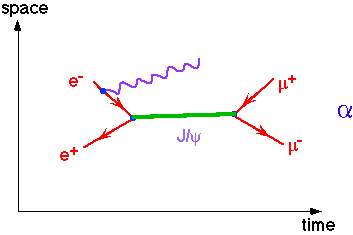Resonances

When a system of two interacting particles have the same quantum numbers (invariant mass, spin, charge, …) as a particle, they can form that particle as a resonance. The maximum total coupling strength for a given particle is the unitarity limit, so if the resonant particle can couple to more than one final state, then the branching ratios and cross sections for a particular final state are proportional to the coupling strength.

(The Breit-Wigner energy distribution is simply the Fourier transform of an exponential decay time distribution.)

p+p total cross section: D++(1236)Mass: M = 1232 MeV      Full Width: G=120 MeV

Spin: 3/2

Branching Ratio : BR(D++Æ p+p)=99.5%

p+p Æp+p cross section at peak of resonance:e+ e- -->r0,w0 -->p+ p-        (F&H Figure 10.20)

 r0 w0 h0 Mass: 769 MeV 782 MeV 547 MeV Full Width: G=151 MeV 8.4 MeV 0.0012 MeV SpinParity: 1- 1- 0- Branching Ratios : BR(p+ p-) ~100% 2.2% - BR(e + e-) 4.5x10-5 7.2x10-5 10-10 (theory) |Fp|2at peak (if no      interference) 38 1.3 -

The non-resonant QED (Quantum Electrodynamic) cross section for the production of pions iswhere p/3s is the cross section for massless point pions, b3 is the threshold dependence for massive pions (139.6 MeV/c2), and Fp2 is the form factor for real composite pions.

The h meson is not observed because it is a pseudoscalar meson and it cannot mix with a single virtual photon to mediate a decay into an electron-positron pair.

e+ e- -->f -->K+ K-        (F&H Figure 10.21)

Mass: M(f) = 1019.4 MeV       M(K±)=493.7 MeV

Full Width: G=4.41 MeV

Spin: 1-

Branching Ratio : BR(f--> e +e-)=3.1x10-4,

BR(f--> K +K-)=50%

Cross section: speak=2.2mb     (xphase space)

e+ e- --> J/y Æhadrons        (F&H Figure 10.23)

Mass: M(J/y) = 3097 MeV

Full Width: G=0.07 MeV

Spin: 1-

Branching Ratio : BR(J/y --> e +e-)=6.9%,